# Working with DataFrames in Julia

A Data frame is a two-dimensional data structure that resembles a table, where the columns represent variables and rows contain values for those variables. It is mutable and can hold various data types.

Julia is a high performance, dynamic programming language which has a high-level syntax. The DataFrame package in Julia gives an ability to create and use data frames to manipulate data for data science and machine learning purposes. To do this, you must gain enough knowledge about data frames in Julia.

To know more about the Data Frame package, visit official documentation.

#### Creating a data frame

Data frame package for Julia must be intstalled in order to use data frames. Type the following commands in the Julia command prompt and click enter to install the data frame package:

```using Pkg

The end of the installation process should appear like in the image shown below: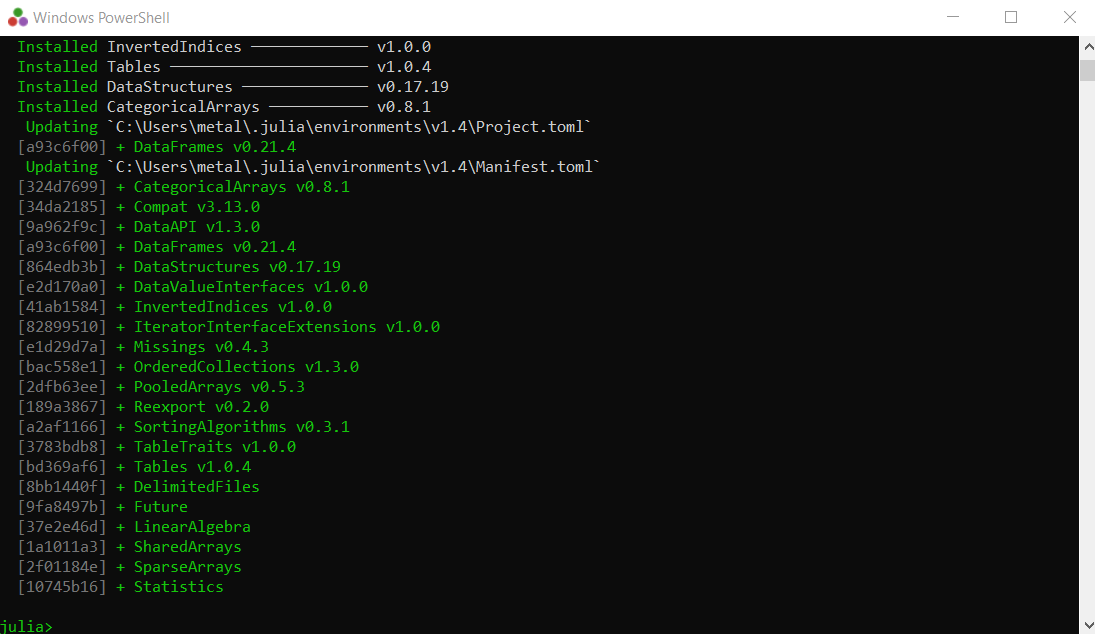Now that you have installed the data frame package, you can create a data frame in various ways.

You can simply create a data frame using the `DataFrame()` function. You can mention the columns and their values in between the brackets of the `DataFrame()` function as the argument and run it as shown below. Before this you have to tell Julia that you are going to use data frames by using the command ‘using DataFrames‘.

Example 1:

 `# Creating a data frame ` `using DataFrames ` ` `  `df ``=` `DataFrame(A ``=` `1``:``5``, B ``=` `[``"A"``, ``"E"``, ``"I"``, ``"O"``, ``"U"``], ` `                        ``C ``=` `[``"A"``, ``"B"``, ``"C"``, ``"D"``, ``"E"``]) `

Output: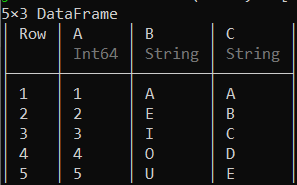You can also create an empty data frame and fill in the columns separately as shown below.

Example 2:

 `# Creating a data frame by adding columns separately ` `df ``=` `Dataframe() ` ` `  `df.C ``=` `1``:``5` `df.D ``=` `[``"A"``, ``"E"``, ``"I"``, ``"O"``, ``"U"``] ` `df `

Output: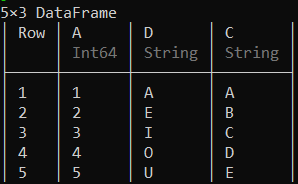Another method for creating a data frame is to add rows to an empty data frame one by one with the `push!()` function. You will have to declare the type of the columns before inserting the rows.

Example 3:

 `# Creating a data frame by adding rows separately ` `df ``=` `Dataframe(E ``=` `Int``[], F ``=` `String[]) ` ` `  `push!(df, (``1``, ``"A"``)) ` `push!(df, (``2``, ``"E"``)) ` `push!(df, (``3``, ``"I"``)) ` `push!(df, (``4``, ``"O"``)) ` `push!(df, (``5``, ``"U"``)) ` `df `

Output: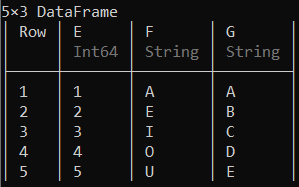#### Accessing Rows and Columns

Now that you have created the data frame, it can be explored. We will be using the data frame which was created first with the columns ‘A’, ‘B’ and ‘C’. To access the first or last few rows you can use the first(DataFrame, rows) or last(DataFrame, rows) functions respectively where ‘rows’ represent the number of rows you want to access.

Example 1:

 `# Selecting the first two rows of the data frame ` `first(df, ``2``) `

Output: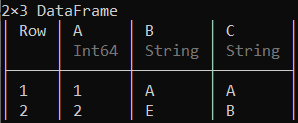Example 2:

 `# Selecting the last two rows of the data frame ` `last(df, ``2``)`

Output: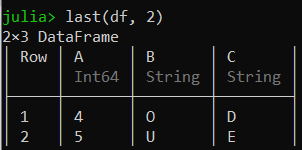To select specific number of rows or columns, you can mention the index numbers or variables of the rows or columns you want to access respectively in ‘df[:, :]’ as shown below.

Example 3:

 `# Selecting the 3rd row of the data frame ` `df[:``3``, :]`

Output: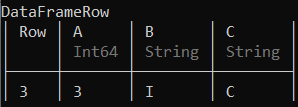Example 4:

 `# Selecting column 'B' of the data frame ` `df[:, [:B]]`

Output: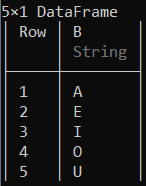#### Creating a subset of the data frame

To create a subset of the data frame with specific columns and number of rows you can use the `select()` function as shown below:
Example 1:

 `# Creating a subset with column 'B' and  ` `# first 3 rows of the data frame ` `first(select(df, :B ), ``3``) `

Output: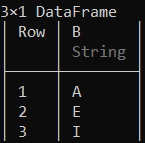You can also create a subset excluding a specific column with the select() as shown below.

Example 2:

 `# Creating a subset with first 4 rows and  ` `# excluding column 'B' of the data frame ` `first(select(df, Not(:B)), ``4``) `

Output: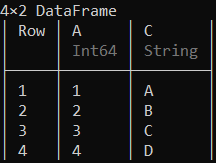A subset of a data frame can also be easily created with specific rows and columns as shown below.

Example 3:

 `# Creating a subset with 2nd, 3rd and 4th rows ` `# and 'B', 'C' columns of the data frame ` `df[``2``:``4``, [:B, :C]] `

Output: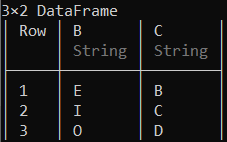#### Modifying content of a data frame

Data can be replaced with some other data in a data frame using various functions. To perform replacement operations in a data frame you can simply use the `replace!()` function.
Example 1:

 `# Replacing the number 4 with 7 in the column 'A' ` `replace !(df.A, ``4` `=``> ``7``) ` `df`

Output: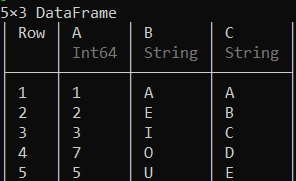Replacement operations on multiple columns can be performed using the broadcasting syntax which creates a subset as shown below.

Example 2:

 `# Replacing the character 'E' with 'None' ` `# in the columns 'B' and 'C' ` `df[:, [:B, :C]] .``=` `ifelse.(df[!, [:B, :C]] .``=``=` `"E"``, ``"None"``, df[!, [:B, :C]]) ` `df `

Output: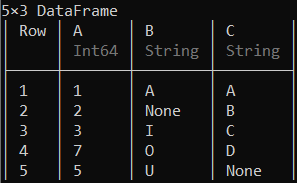Replacement operation for the full data frame can be performed as shown below.

Example 3:

 `# Replacing the character 'A' with 'E' in the data frame ` `df .``=` `ifelse.(df .``=``=` `"A"``, ``"E"``, df) `

Output: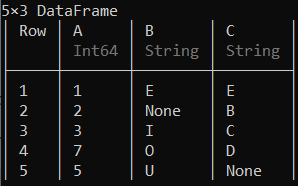Here every ‘A’ is replaced with ‘E.

#### Adding extra rows and columns to the data frame

New rows can be added to the end of a data frame by using `push!()` function.
Example 1:

 `# Adding a new column to the end of the data frame ` `push !(df, [``6` `"None"` `"F"``]) `

Output: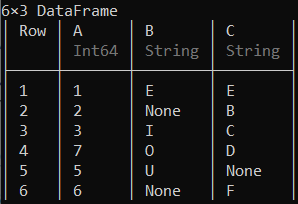A column can be added to a specific position in a data frame by using the `insert!()` function.

Example 2:

 `# Adding a new column to the 3rd position  ` `# of the data frame ` `insert !(df, ``3``, [``"Q"``, ``"W"``, ``"E"``, ``"R"``, ``"T"``, ``"Y"``], :D) `

Output: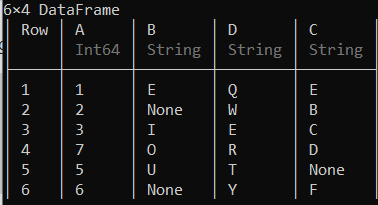You can also add a column by creating an array of elements you want in the column and add it to the end of the data frame as shown below.

Example 3:

 `# Adding a new column in the  ` `# last postion of the data frame ` `arr ``=` `[``2``, ``3``, ``5``, ``7``, ``11``, ``13``] ` `df[:E] ``=` `arr ` `df `

Output: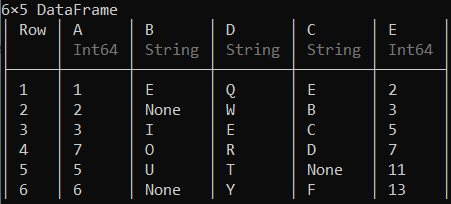#### Delete rows and columns in a data frame

A specific row from a data frame can be deleted using the `deleterows!()` function.

Example 1:

 `# Deleting the 4th row of the data frame ` `deleterows !(df, ``4``) `

Output: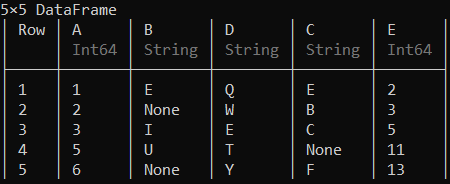A column can be deleted using the `deletecols!()` function.

Example 2:

 `# Deleting the 3rd coloumn of the data frame ` `deletecols !(df, ``3``) `

Output: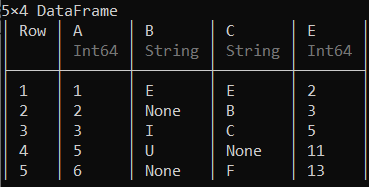Here the column ‘D’, which is the 3rd column had been deleted.

#### Merging of Data frames

Multiple data frames are created here to represent the implementation of merging operations.

Example 1:

 `# Creating new data frames ` `df2 ``=` `DataFrame(F ``=``[``"A"``, ``"E"``, ``"I"``, ``"O"``, ``"U"``],  ` `                ``G ``=``[``"G"``, ``"E"``, ``"E"``, ``"K"``, ``"S"``]) ` `df4 ``=` `DataFrame(H ``=``[``"G"``, ``"R"``, ``"E"``, ``"A"``, ``"T"``]) `

Output: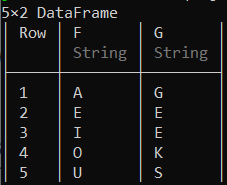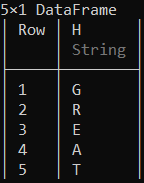You can merge these two new data frames with the first one using the concatenating function hcat(). This function merges data frames horizontally, note that all of the data frames should contain the same number of rows when merging horizontally.

Example 2:

 `# Merging the created data frames horizontally ` `hcat(df, df2, df4) `

Output: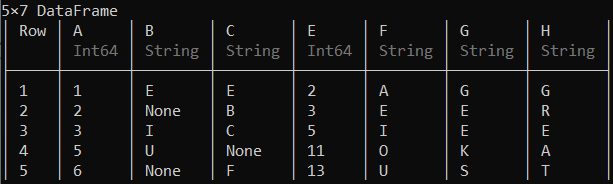`vcat()` concatenating function can be used to merge data frames vertically, hence to represent that data frames with the same number and names of the columns as the first data frame are created.

Example 3:

 `# Creating new data frames ` `df3 ``=` `DataFrame(A ``=` `7``, B ``=``"O"``, C ``=``"G"``, E ``=` `17``) ` `df5 ``=` `DataFrame(A ``=` `8``, B ``=``"None"``, C ``=``"H"``, E ``=` `19``) ` ` `  `# Merging the created dataframes vertically ` `vcat(df, df3, df5) `

Output: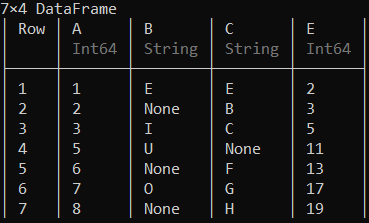Basic data frames with manually entered data were used here. Many more operations can be done with many functions for CSV files containing huge amounts of data for analysis.

My Personal Notes arrow_drop_upCheck out this Author's contributed articles.

If you like GeeksforGeeks and would like to contribute, you can also write an article using contribute.geeksforgeeks.org or mail your article to contribute@geeksforgeeks.org. See your article appearing on the GeeksforGeeks main page and help other Geeks.

Please Improve this article if you find anything incorrect by clicking on the "Improve Article" button below.

Article Tags :

Be the First to upvote.

Please write to us at contribute@geeksforgeeks.org to report any issue with the above content.